﻿ 融入学习者模型在线学习资源协同过滤推荐方法
«上一篇文章快速检索 高级检索

 智能系统学报2021, Vol. 15Issue (6): 1117-1125  DOI: 10.11992/tis.2020090050

### 引用本文LIU Fang, TIAN Feng, LI Xin, et al. A collaborative filtering recommendation method for online learning resources incorporating the learner model[J]. CAAI Transactions on Intelligent Systems, 2021, 15(6): 1117-1125. DOI: 10.11992/tis.202009005.### 文章历史

1. 东北石油大学 计算机与信息技术学院，黑龙江 大庆 163318;
2. 讷河市第一中学，黑龙江 讷河 161300

A collaborative filtering recommendation method for online learning resources incorporating the learner model
LIU Fang 1, TIAN Feng 1, LI Xin 2,  LIN Lin 1
1. School of Computer and Information Technology, Northeast Petroleum University, Daqing 163318, China;
2. Nehe No. 1 Middle School, Nehe 161300,China
Abstract: Online education exhibits the problem of “information loss”. At the same time, traditional information recommendation methods often ignore the characteristics of learners, i.e., the main body of education. Based on the theory of education and teaching as well as the relevant data of learners on the online education platform, this paper constructs a learner model suitable for personalized recommendations for online learning resources. Based on the collaborative filtering recommendation method, the static and dynamic features of the learner model are integrated, with the aim to improve the collaborative filtering method, thereby establishing a collaborative filtering recommendation method for online learning resources incorporating the learner model. The real learning and behavior records of students taking the C programming course in the Northeast Petroleum University starting from March 2020 to July 2020 were selected as the dataset to conduct experiments and evaluations on the proposed research method. The comparative test shows that the performance of the proposed method is better than that of the comparative method.
Key words: learner models    online learning resources    collaborative filtering    personalized learning    learning resources recommendation    learning style characteristics    cognitive level characteristics    interest preference characteristics

1 多维度学习者模型构建

1.1 数据采集

1.2 学习风格的特征表示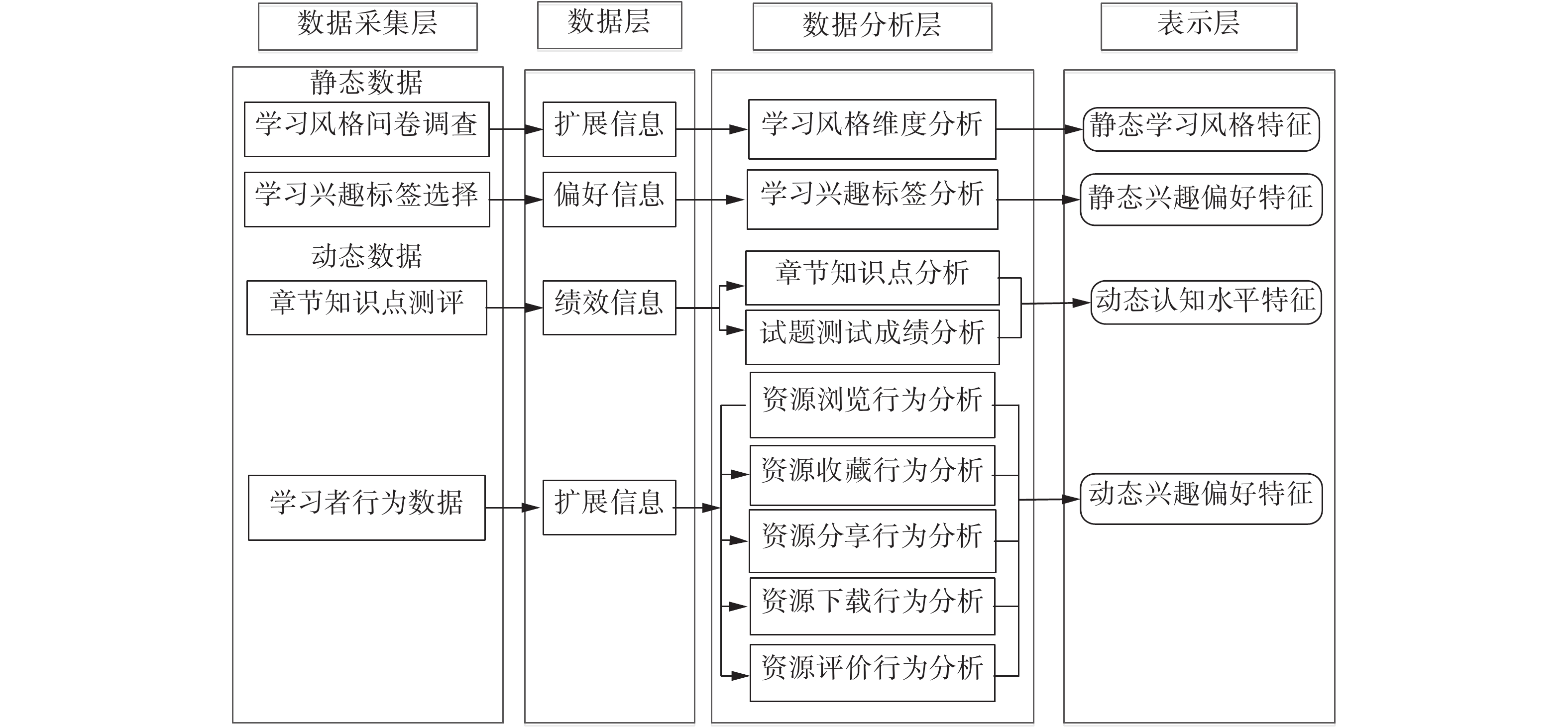Download: 图 1 学习者模型构建过程 Fig. 1 Building process of learner model

1）以四元组<LiVi>(i={1,2,3,4})的形式表示学习风格量化结果，Li表示ILSQ的4个维度；Vi表示在Li维度下的学习风格倾向的量化数值，其形式化定义为

 $\begin{array}{l}{{L}}_{\rm{S}}=\left\{(\langle {L}_{\text{1}},{V}_{\text{1}}\rangle ,\langle {L}_{\text{2}},{V}_{\text{2}}\rangle ,\langle {L}_{\text{3}},{V}_{\text{3}}\rangle ,\right.\\ \left. \quad\quad \langle {L}_{\text{4}},{V}_{\text{4}}\rangle )|{V}_{i}\in \left[{-1}\text{，}\text{1}\right]\right\}\end{array}$

2）学习者填写ILSQ量表时，共44道题，每题包含两个选项A和B，答题结果的值定义为Pj，其中j表示题号；

3）根据Pj的结果筛选处理，分类累加，最后的累加结果用ab表示；

4）对ab值的大小进行判断，如果a>b，则Vi=(ab)a；如果a<b，则Vi=(ba)a

5）学习风格特征的测试结果四元组LS则为学习者的学习风格特征量化结果。

1.3 兴趣偏好的特征表示

1）学习者行为分类及权重计算表 1 学习者行为分类及权重分布 Tab.1 Learner behavior classification and weight distribution

2）学习者−学习资源评分矩阵构建

 ${{\boldsymbol{P}}_{m \times n}} = \left[ {\begin{array}{*{20}{c}} {{s_{{\text{11}}}}}&{{s_{{\text{12}}}}}&{\cdots}&{{s_{{\text{1}}n}}} \\ {{s_{{\text{21}}}}}&{{s_{{\text{22}}}}}&{\cdots}&{{s_{{\text{2}}n}}}\\ \vdots & \vdots &{}& \vdots \\ {{s_{m{\text{1}}}}}&{{s_{m{\text{2}}}}}&{\cdots}&{{s_{mn}}} \end{array}} \right]$

Pm×n矩阵中的每个值都表示学习者um对资源in的行为权重，如果sui=0，那么说明学习者uj并未对ik产生任何行为，如果矩阵元素全为0，则说明学习者uj并没有开始学习。

3）学习资源−学习标签矩阵构建

 ${{\boldsymbol{Q}}_{{{n}} \times {{l}}}} = \left[ {\begin{array}{*{20}{c}} {{r_{{\text{11}}}}}&{{r_{{\text{12}}}}}&{\cdots}&{{r_{{\text{1}}l}}} \\ {{r_{{\text{21}}}}}&{{r_{{\text{22}}}}}&{\cdots}&{{r_{{\text{2}}l}}} \\ \vdots & \vdots &{}& \vdots \\ {{r_{n{\text{1}}}}}&{{r_{n{\text{2}}}}}&{\cdots}&{{r_{nl}}} \end{array}} \right]$

Qn×l矩阵中的元素rjk表示资源ij是否拥有标签tkrjk=1表示标签tk标注了资源ijrjk=0表示未被标注，因此矩阵Qn×l是一个由0和1构成的矩阵。

 ${{\boldsymbol{T}}_{m \times l}} = \left[ {\begin{array}{*{20}{c}} {{g_{{\text{11}}}}}&{{g_{{\text{12}}}}}&{\cdots}&{{g_{{\text{1l}}}}} \\ {{g_{{\text{21}}}}}&{{g_{{\text{22}}}}}&{\cdots}&{{g_{{\text{2}}l}}} \\ \vdots & \vdots &{}& \vdots \\ {{g_{m{\text{1}}}}}&{{g_{m{\text{2}}}}}&{\cdots}&{{g_{ml}}} \end{array}} \right]$

4）学习者动态兴趣偏好行为特征表示

 $F_{u{t_k}}^{{\text{op}}} = \exp \left(\frac{{{g_{uk}}}}{{{v_{u{t_k}}}}} - \lambda \right),\quad1 \leqslant k \leqslant l$

5）时间因素调整动态兴趣偏好特征的偏移

 $F_{u{t_k}}^{{\text{time}}} = \theta + (1 - \theta )\exp [ - ({t_{{\text{now}}}} - {t_{u{t_k}}})],\quad1 \leqslant k \leqslant 1$

 ${F}_{u{t}_{k}}^{}={F}_{u{t}_{k}}^{\text{op}}{F}_{u{t}_{k}}^{\text{time}},\quad1\leqslant k\leqslant l$
1.4 认知水平的特征表示

 $K_L= \{ ({k_1},{l_1}{\text{),}} ({k_2},{l_2}{\text{),}}\cdots ,{\text{(}}{k_i},{l_i}{\text{)}}, \cdots ,{\text{(}}{k_n},{l_n}{\text{)\} }}$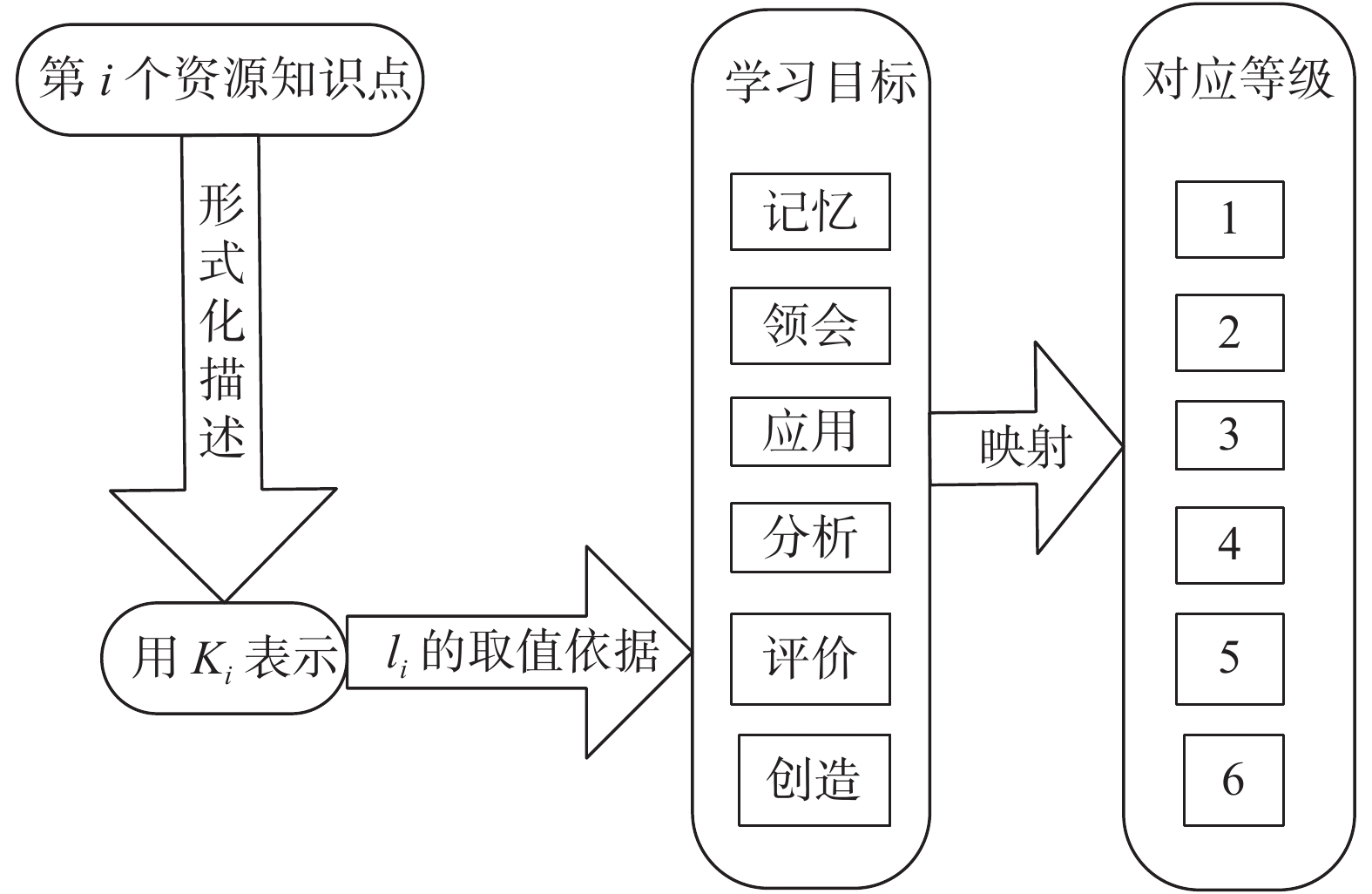Download: 图 2 学习资源知识点掌握程度的表示方法 Fig. 2 Representation method of learning resources knowledge points master degree
2 融合学习者模型的协同过滤改进

2.1 基于协同过滤的在线学习资源推荐

2.2 融合学习者静态特征的协同过滤推荐改进

1）静态兴趣偏好特征相似度计算

 ${\text{sim}}{(u_m,u_n)_{{\text{interest}}}} = \frac{k}{{q + p - k}}$

2）学习风格特征相似度计算

 $d(S_{m},S_{n})_{{\rm{style}}} = \sqrt {\sum\limits_{k = 1}^4 {(\mathop S\nolimits_{{m}}^{{{{t}}_{{k}}}} - \mathop S\nolimits_{{n}}^{{{{t}}_{{k}}}} ){}^2} }$

umun的学习风格相似度计算公式为

 ${\text{sim}}{(u_m,u_n)_{{\text{style}}}} = \frac{1}{{1 + d(S_m,S_n)}}$

3）静态综合特征相似度计算

 $\begin{gathered} {\text{sim}}{(u_m,u_n)_{{\text{static}}}} = \\ \alpha {\text{sim}}(u_m,u_n)_{\text{interest}} + {\text{(1 }}-\alpha {\text{)sim}}{(u_m,u_n)_{{\text{style}}}} \\ \end{gathered}$
2.3 融合学习者动态特征的协同过滤推荐改进

1）融合行为特征和时间权重特征的兴趣偏好相似度计算

 $\begin{array}{l} \quad\quad\quad{\rm{sim}}{({u_m},{u_n})_{{\rm{interest}}}}_{{\rm{\_updata}}} = \\ \frac{{\displaystyle\sum\limits_{{t_i} \in {T_{mn}}} {({F_{m,{t_i}}} - {\overline{F}_{m}} ) \times ({F_{n,{t_i}}} - {\overline{F}_{n}} )} }}{{\sqrt {\displaystyle\sum\limits_{{t_i} \in {T_{{{mn}}}}} {{{({F_{m,{t_i}}} - {\overline{F}_{m}} )}^2}} } \times \sqrt {\displaystyle\sum\limits_{{t_i} \in {T_{{{mn}}}}} {{{({F_{m,{t_i}}} - {\overline{F}_{n}} )}^2}} } }} \end{array}$

2）融合认知水平特征的相似度计算

 ${\text{sim}}{(u_m{\text{,}}u_n)_{{\text{level}}}} = \frac{{{u_m} \cdot {u_n}}}{{\left| {{u_m}} \right| \cdot \left| {{u_n}} \right|}}\frac{{\displaystyle\sum\limits_k {h{{_j^{}}_{,{u_m}}}{h_{j,{u_n}}}} }}{{\sqrt {\displaystyle\sum\limits_k {h{{_j^2}_{,{u_m}}}} } \sqrt {\displaystyle\sum\limits_k {h{{_j^2}_{,{u_n}}}} } }}$

3）学习者动态综合特征相似度计算

 $\begin{gathered} \quad\quad\quad\quad\quad\quad{\text{sim}}{(u_m{\text{,}}u_n)_{{\text{static}}}} = \hfill \\ \beta {\text{sim}}(u_m{\text{,}}u_n)_{\text{interest\_updata}} + {\text{(1}}-\beta {\text{)sim}}{(u_m{\text{,}}u_n)_{{\text{style\_updata}}}} \hfill \\ \end{gathered}$
3 实验结果与分析 3.1 实验数据集

3.2 评价标准

 $P = \sum_{u \in U} {{\text{|}}{\boldsymbol{R}}(u) \cap {{\boldsymbol{T}}}(u){\text{|}}} /\sum_{u \in U} {{\text{|}}{\boldsymbol{R}}(u)} |$

 $R = \sum_{u \in U} {{\text{|}}{\boldsymbol{R}}{\text{(}}u{\text{)}} \cap {\boldsymbol{T}}{\text{(}}u{\text{)|}}} /\sum_{u \in U} {{\text{|}}{\boldsymbol{T}}{\text{(}}u{\text{)}}} |$

 $F_1 = 2 \times {\text{AP}} \times {\text{AR/(AP}} + {\text{AR)}}$
3.3 实验结果与分析

1）融合学习者静态特征实验分析

2）融合学习者动态特征实验分析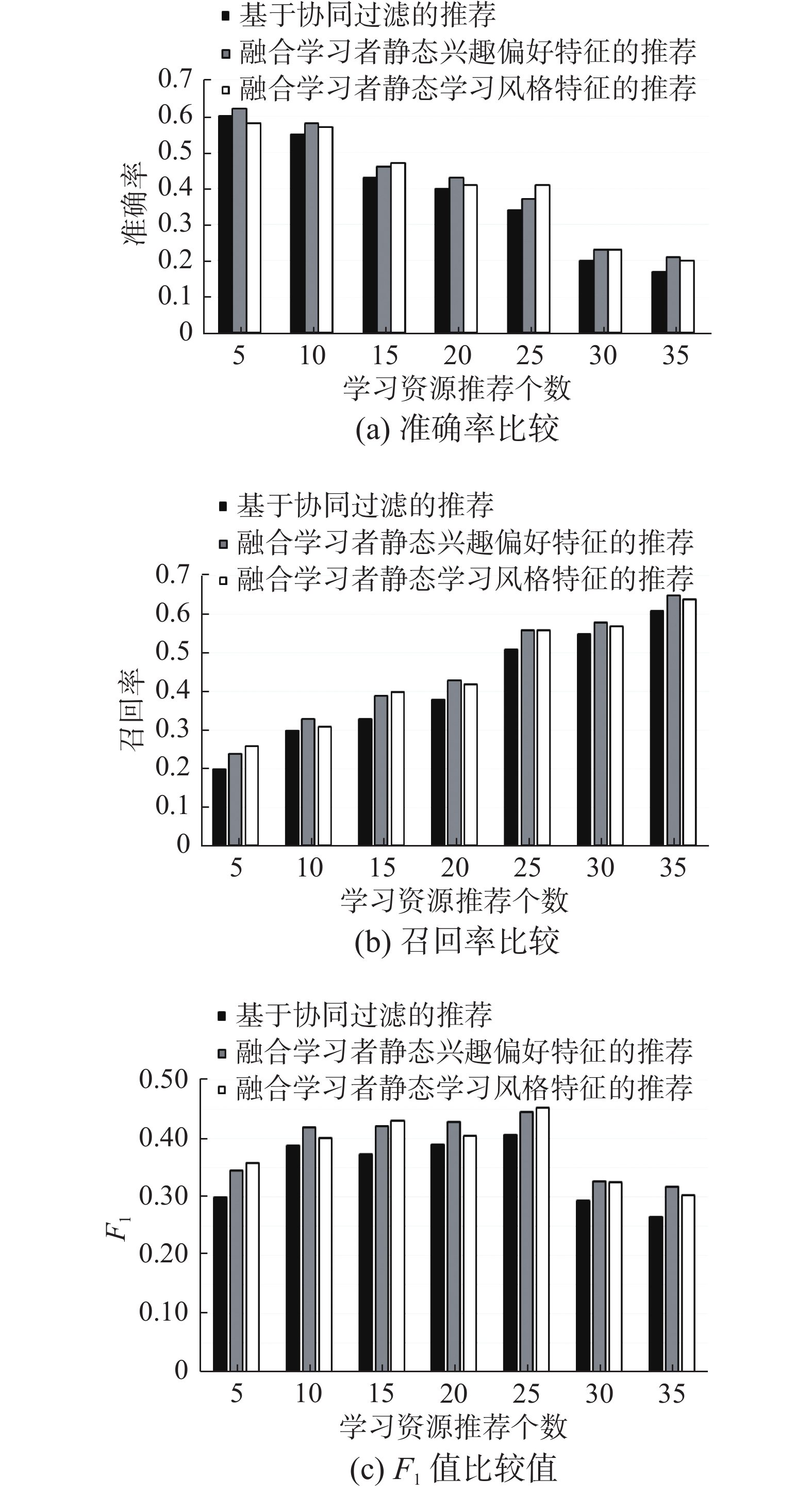Download: 图 3 融合学习者各项静态特征的推荐性能 Fig. 3 Recommended performance of integrating learners’ various static characteristics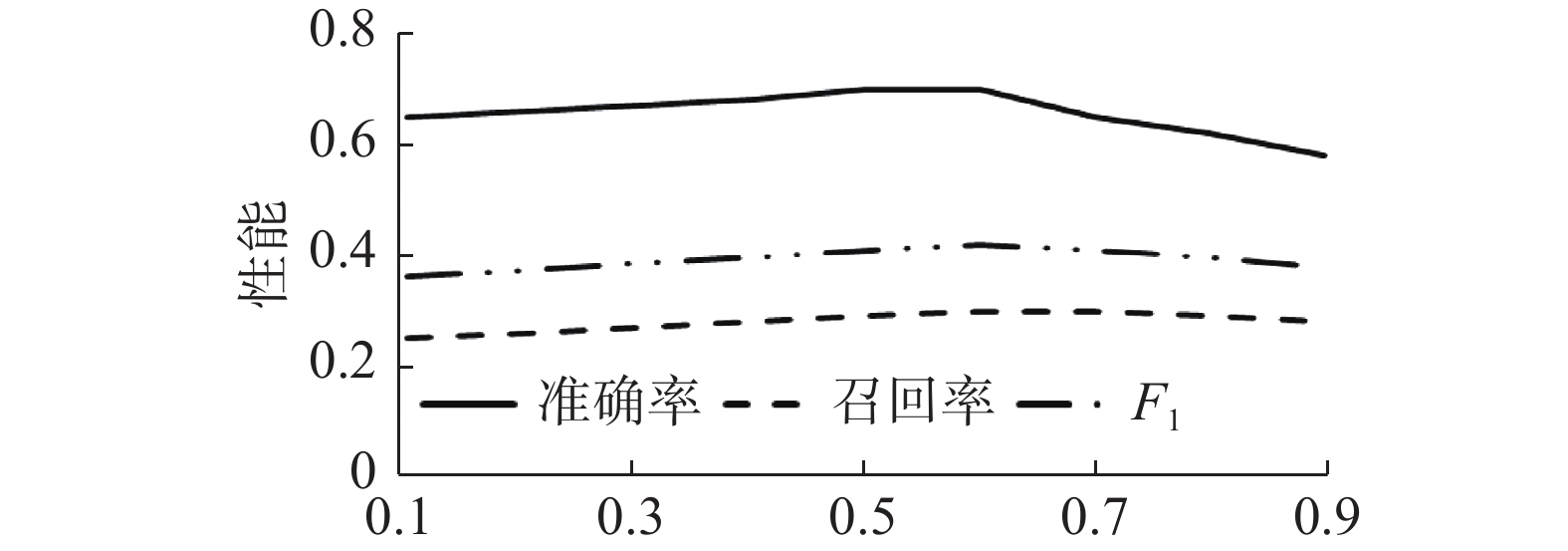Download: 图 4 静态特征融合参数选择比较 Fig. 4 Static feature fusion parameter selection comparison

3）综合对比分析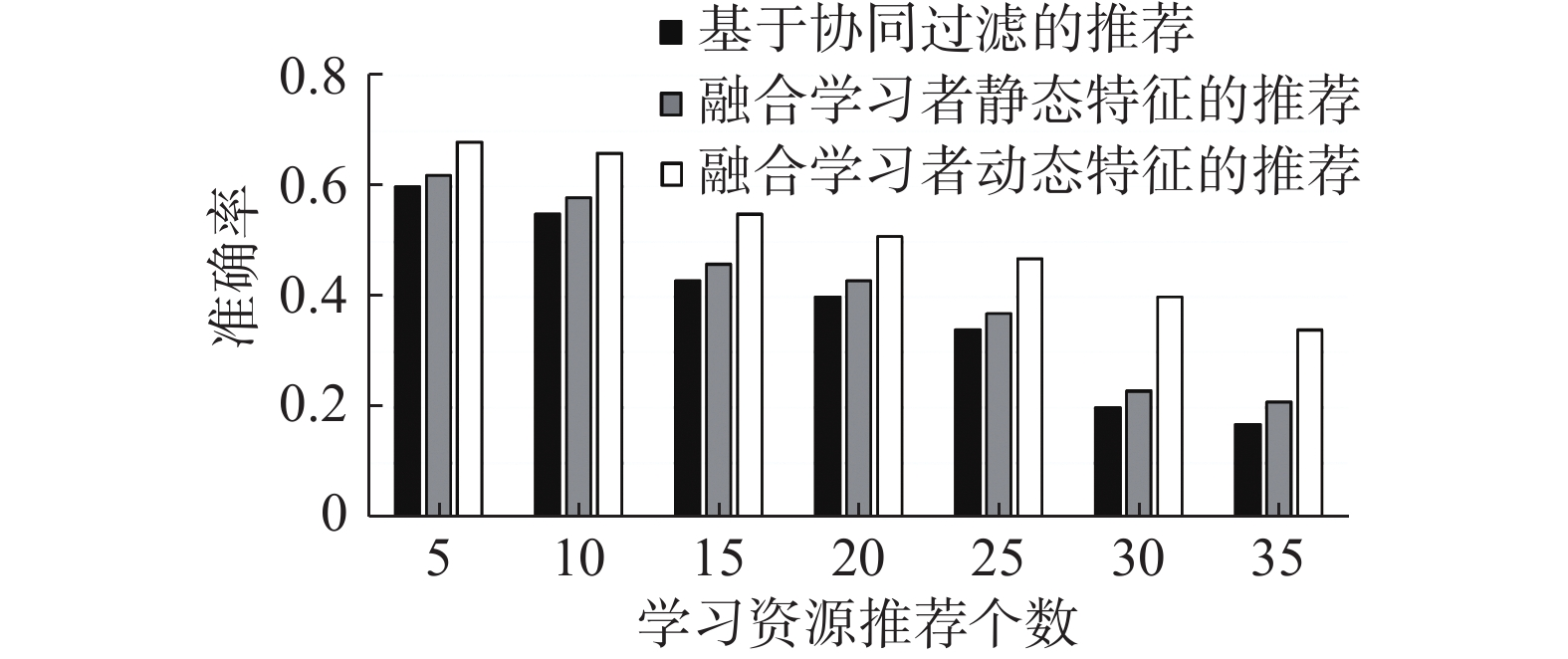Download: 图 5 不同推荐方法的准确率比较 Fig. 5 Accuracy comparison of different recommended methods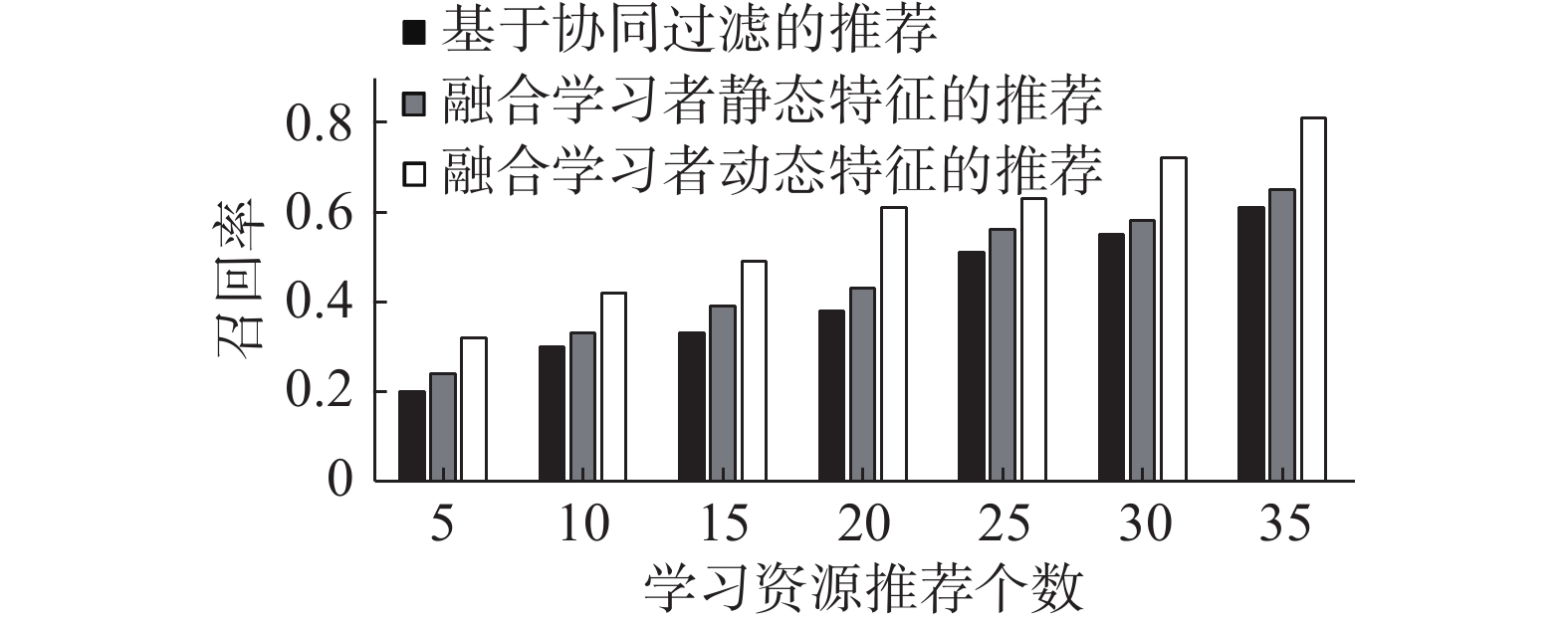Download: 图 6 不同推荐方法的召回率比较 Fig. 6 Recall rate comparison of different recommended methods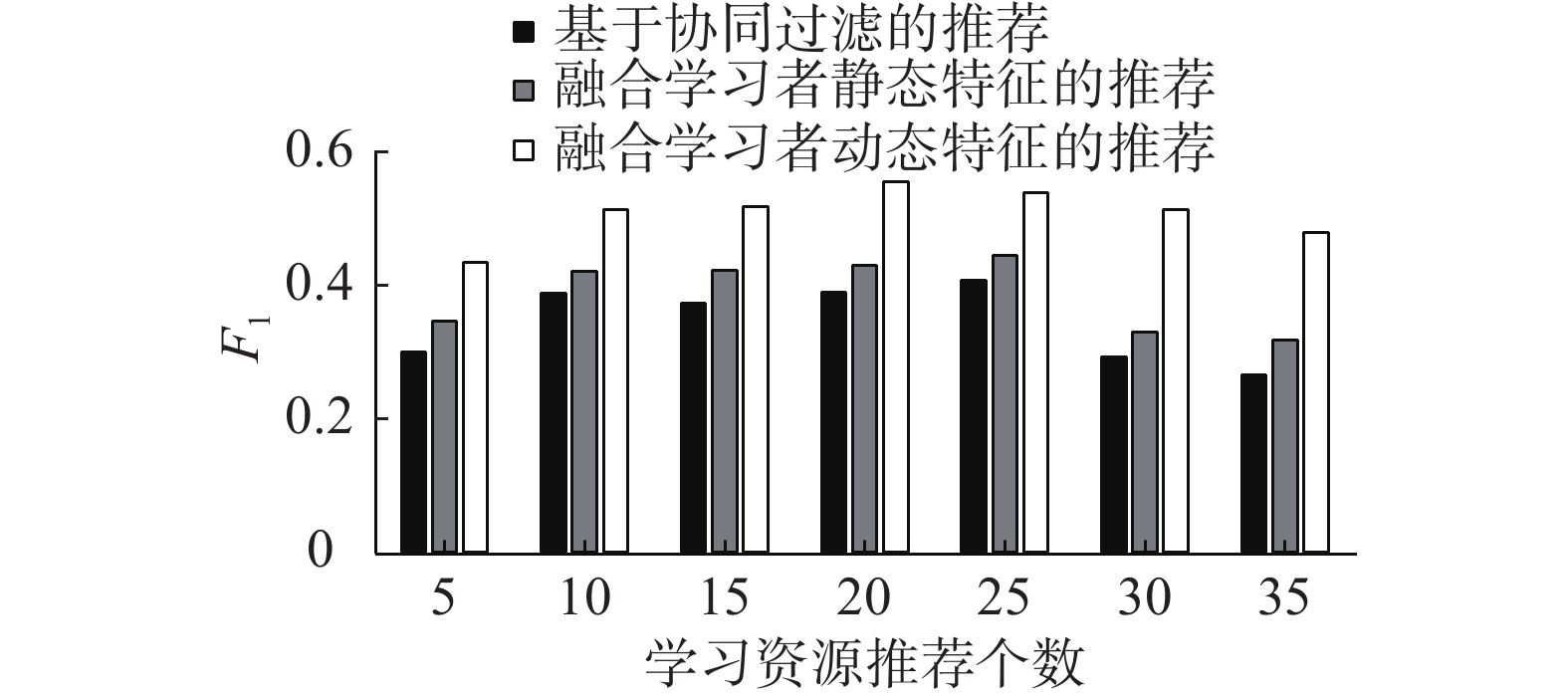Download: 图 7 不同推荐方法F1值比较 Fig. 7 F1 value comparison of different recommended methods

4 结束语

  中国互联网络信息中心(CNNIC). 第45次中国互联网络发展状况统计报告[R]. 北京: 中国互联网络信息中心(CNNIC), 2020: 4. (0)  AL-SHAMRI M Y H. Power coefficient as a similarity measure for memory-based collaborative recommender systems[J]. Expert systems with applications, 2014, 41(13): 5680-5688. DOI:10.1016/j.eswa.2014.03.025 (0)  NAJAFABADI M K, MOHAMED A, ONN C W. Animpact of time and item influencer in collaborative filtering recommendations using graph-based model[J]. Information processing & management, 2019, 56(3): 526-540. (0)  WANG Yong, DENG Jiangzhou, GAO J, et al. A hybrid user similarity model for collaborative filtering[J]. Information sciences, 2017, 418−419: 102-118. (0)  JIANG Shan, FANG S C, AN Qi, et al. A sub-one quasi-norm-based similarity measure for collaborative filtering in recommender systems[J]. Information sciences, 2019, 487: 142-155. DOI:10.1016/j.ins.2019.03.011 (0)  MU Yi, XIAO Nianhao, TANG Ruichun, et al. An efficient similarity measure for collaborative filtering[J]. Procedia computer science, 2019, 147: 416-421. DOI:10.1016/j.procs.2019.01.258 (0)  WANG T I, TSAI K H, LEE M C, et al. Personalized learning objects recommendation based on the semantic-aware discovery and the learner preference pattern[J]. Educational technology & society, 2007, 10(3): 84-105. (0)  SEGAL A, KATZIR Z, GAL Y, et al. EduRank: a collaborative filtering approach to personalization in E-learning[C]//Proceedings of the 7th International Conference on Educational Data Mining. London, UK, 2014: 68−74. (0)  ZHANG Fuzheng, YUAN N J, LIAN Defu, et al. Collaborative knowledge base embedding for recommender systems[C]//Proceedings of the 22nd ACM SIGKDD International Conference on Knowledge Discovery and Data Mining. San Francisco, USA, 2016: 353−362. (0)  KLAŠNJA-MILIĆEVIĆ A, IVANOVIĆ M, VESIN B, et al. Enhancing e-learning systems with personalized recommendation based on collaborative tagging techniques[J]. Applied intelligence, 2018, 48(6): 1519-1535. DOI:10.1007/s10489-017-1051-8 (0)  BAKER R S J D, CORBETT A T, ALEVEN V. More accurate student modeling through contextual estimation of slip and guess probabilities in Bayesian knowledge tracing[C]//Proceedings of the 9th International Conference on Intelligent Tutoring Systems. Montreal, Canada, 2008. (0)  SALEHI M. Application of implicit and explicit attribute based collaborative filtering and BIDE for learning resource recommendation[J]. Data & knowledge engineering, 2013, 87: 130-145. (0)  KURILOVAS E, SERIKOVIENE S, VUORIKARI R. Expert centred vs learner centred approach for evaluating quality and reusability of learning objects[J]. Computers in human behavior, 2014, 30: 526-534. DOI:10.1016/j.chb.2013.06.047 (0)  现代远程教育技术标准化委员会. CELTS-11, 学习者模型规范[S]. 现代远程教育技术标准化委员会, 2000: 11. (0)  COSTA R D, SOUZA G F, VALENTIM R A M, et al. The theory of learning styles applied to distance learning[J]. Cognitive systems research, 2020, 64: 134-145. DOI:10.1016/j.cogsys.2020.08.004 (0)  ARIEVITCH I M. Reprint of: the vision of Developmental Teaching and Learning and Bloom's Taxonomy of educational objectives[J]. Learning, culture and social interaction, 2020, 27: 100473. DOI:10.1016/j.lcsi.2020.100473 (0)  ZLATKOVIC D, DENIC N, PETROVIC M, et al. Analysis of adaptive e-learning systems with adjustment of Felder-Silverman model in a Moodle DLS[J]. Computer applications in engineering education, 2020, 28(4): 803-813. DOI:10.1002/cae.22251 (0)  DASCALU M I, BODEA C N, MOLDOVEANU A, et al. A recommender agent based on learning styles for better virtual collaborative learning experiences[J]. Computers in human behavior, 2015, 45: 243-253. DOI:10.1016/j.chb.2014.12.027 (0)  GONZÁLEZ G, LÓPEZ B, DE LA ROSA J L. A multi-agent smart user model for cross-domain recommender systems[C]//Proceedings of Beyond Personalization 2005: The Next Stage of Recommender Systems Research, International Conference on Intelligent User Interfaces IUI 2005. San Diego, USA, 2005. (0)  谢修娟, 陈永, 李香菊, 等. 融入信任的变权重相似度模型在线学习协同推荐算法[J]. 小型微型计算机系统, 2018, 39(3): 525-528. XIE Xiujuan, CHEN Yong, LI Xiangju, et al. Collaborative recommendation algorithm of online learning based on trust-combined simi-larity model with variable weight[J]. Journal of Chinese computer systems, 2018, 39(3): 525-528. DOI:10.3969/j.issn.1000-1220.2018.03.023 (0)  陈秀明, 刘业政. 多粒度犹豫模糊语言环境下未知权重的多属性群推荐方法[J]. 控制与决策, 2016, 31(9): 1631-1637. CHEN Xiuming, LIU Yezheng. Method of group recommender systems with unknown attribute weights in a multi-granular hesitant fuzzy linguistic term environment[J]. Control and decision, 2016, 31(9): 1631-1637. (0)  KOREN Y. Collaborative filtering with temporal dynamics[C]//Proceedings of the 15th ACM SIGKDD International Conference on Knowledge Discovery and Data Mining. Paris, France, 2009: 89−97. (0)  孙歆, 王永固, 邱飞岳. 基于协同过滤技术的在线学习资源个性化推荐系统研究[J]. 中国远程教育, 2012(8): 78-82. DOI:10.3969/j.issn.1009-458X.2012.08.018 (0)  郑洁, 钱育蓉, 杨兴耀, 等. 基于信任和项目偏好的协调过滤算法[J]. 计算机应用, 2016, 36(10): 2784-2788, 2798. ZHENG Jie, QIAN Yurong, YANG Xingyao, et al. Collaborative filtering algorithm based on trust and item preference[J]. Journal of computer applications, 2016, 36(10): 2784-2788, 2798. DOI:10.11772/j.issn.1001-9081.2016.10.2784 (0)  丁永刚, 张馨, 桑秋侠, 等. 融合学习者社交网络的协同过滤学习资源推荐[J]. 现代教育技术, 2016, 26(2): 108-114. DING Yonggang, ZHANG Xin, SANG Qiuxia, et al. The collaborative filtering recommendation of learning resources combined with learners’ social network[J]. Modern educational technology, 2016, 26(2): 108-114. DOI:10.3969/j.issn.1009-8097.2016.02.016 (0)  刘忠宝, 宋文爱, 孔祥艳, 等. 云环境下学习者建模与学习资源推荐方法研究[J]. 电化教育研究, 2017, 38(7): 58-63. LIU Zhongbao, SONG Wenai, KONG Xiangyan, et al. Research on learner modeling and learning resources recommendation in cloud environment[J]. E-education research, 2017, 38(7): 58-63. (0)